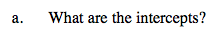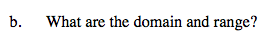### Home > A2C > Chapter 10 > Lesson 10.2.6 > Problem10-148

10-148.To find the y-intercept, substitute 0 for x.

h(0) = −2(9) + 2

To find the x-intercept substitute 0 for h(x).

0 = −2(x + 3)2 + 2

−2(x + 3)2 = −2

(x + 3)2 = 1

x + 3 = ± 1

y-intercept (0, −16)

x-intercept (−4, 0), (−2, 0)Which variable is being squared?

Domain: all real numbers
Range: y ≤ 2